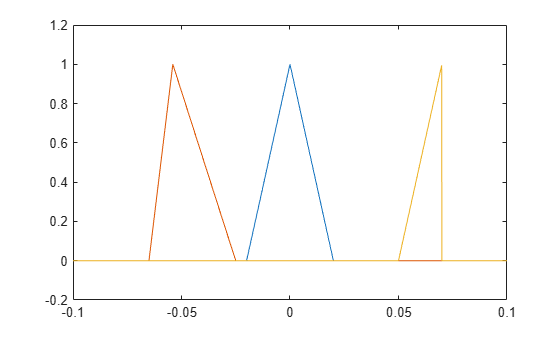# tripuls

Sampled aperiodic triangle

## Syntax

```y = tripuls(T) y = tripuls(T,w) y = tripuls(T,w,s) ```

## Description

`y = tripuls(T)` returns a continuous, aperiodic, symmetric, unity-height triangular pulse at the times indicated in array `T`, centered about `T=0` and with a default width of 1.

`y = tripuls(T,w)` generates a triangular pulse of width `w`.

`y = tripuls(T,w,s)` generates a triangular pulse with skew `s`, where –1 ≤ `s` ≤ 1. When `s` is 0, a symmetric triangular pulse is generated.

## Examples

collapse all

Generate 200 ms of a symmetric triangular pulse with a sample rate of 10 kHz and a width of 40 ms.

```fs = 10e3; t = -0.1:1/fs:0.1; w = 40e-3; x = tripuls(t,w);```

Generate two variations of the same pulse:

• One displaced 45 ms into the past and skewed 45% to the left.

```tpast = -45e-3; spast = -0.45; xpast = tripuls(t-tpast,w,spast);```
• One displaced 60 ms into the future, half as wide, and skewed completely to the right.

```tfutr = 60e-3; sfutr = 1; xfutr = tripuls(t-tfutr,w/2,sfutr);```

Plot the original pulse and the two copies on the same axes.

```plot(t,x,t,xpast,t,xfutr) ylim([-0.2 1.2])```